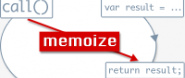## Mootols Memoize 0.3A plugin providing memoization capabilities for all instance methods. Memoizing can be very handy and improve performance greatly if used properly. For more information read here.

### Details

Author
MIke Nelson
Current version
0.3
GitHub
mnelson/mootools-memoize
6828
Category
Native
Tags
Report
GitHub Issues

### Dependencies

• _self_/_current_: core:1.2.4

### How to use

Just add a Memoize mutator to your class implementations after all other methods have been defined. A __memoized hash is created where all return values are stored. Stored values are NOT dependent on the arguments passed so be sure you think before memoizing. If you need to unmemoize a method just call instance.unmemoize(methodName) or instance.unmemoizeAll() to clear the stored value and allow another execution of the method.

```Calculus = new Class({
initialize : function(base_function){
this.base = base_function;
},
setFunction(func){
this.base = func;
},
integral : function(){
// potentially complex code
return result;
},
derivative : function(){
// potentially complex code
return result;
},
Memoize : ['integral', 'derivative']
});

var calc = new Calculus('y = 3x^2 + 2x + 1');

calc.derivative() => (potentially complex code runs) => 'dy/dx = 6x + 2'
calc.derivative() => 'dy/dx = 6x + 2'

calc.setFunction('y = 9x + 2')

calc.derivative() => 'dy/dx = 6x + 2'
calc.unmemoize('derivative') => '6x + 2'
calc.derivative() => (potentially complex code runs) => 'dy/dx = 9'

calc.unmemoizeAll();

calc.derivative() => (potentially complex code runs) => 'dy/dx = 9'
```

### Discuss

A note on comments here: These comments are moderated. No comments will show up until they are approved. Comments that are not productive (i.e. inflammatory, rude, etc) will not be approved.

Found a bug in this plugin? Please report it this repository's Github Issues.